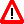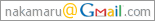# imgTeX

imgTeX is yet another math-on-the-web solution, which works in a similar manner to mimeTeX or others discussed on the mimeTeX web page. imgTeX is based on dvipng and FastCGI and has a simple on-memory cache mechanism. These characteristics enables fast and clear rendering.

The following is a result for the example in Usage, rendered now (note: could be a cached result).

Here are some examples. Both math:, and displaymath:can be used.

You can also write more complicated equations:You can also use imgTeX interactively on Interactive imgTeX.

## News

• Jan 10, 2012: added dvipng options "-bg Transparent" and "--truecolor" for generating transparent png images.
• Oct 10, 2009: added some description for avoiding vulnerability with loose texmf.cnf settings.
• Sep 28, 2007: added Customization.
• Jan 23, 2005: modified options for dvipng a bit.
• Jan 21, 2005: PukiWiki plugin is added.
• Jan 5, 2005: fixed a bug which losts '+' in the equation.
• Jan 4, 2005: added Interactive imgTeX.

## Usage

In your HTML file, you can write as follows:

<blockquote>
<p>Here are some examples. Both math:
<img src="imgtex.fcgi?{$f(x)=\int_{-\infty}^xe^{-t^2}dt$}" style="vertical-align:middle" />,
and displaymath:
<img src="imgtex.fcgi?{$f(x)=\int_{-\infty}^xe^{-t^2}dt$}" style="vertical-align:middle" />
can be used.
</p>
<p>You can also write more complicated equations:</p>
<div style="text-align:center">
<img src="imgtex.fcgi?[res=300]{
\begin{equation*}
A = \begin{pmatrix}
a_{11} & \ldots & a_{1n} \\
\vdots & \ddots & \vdots \\
a_{m1} & \ldots & a_{mn}
\end{pmatrix}
\end{equation*}
}" />
</div>
<div style="text-align:center">
<img src="imgtex.fcgi?[res=200]{
$\frac{\pi}{2} = \left( \int_{0}^{\infty} \frac{\sin x}{\sqrt{x}} dx \right)^2 = \sum_{k=0}^{\infty} \frac{(2k)!}{2^{2k}(k!)^2} \frac{1}{2k+1} = \prod_{k=1}^{\infty} \frac{4k^2}{4k^2 - 1}$
}" />
</div>
</blockquote>

where res is the resolution for dvipng's "-D" option. The above examples are snipped from mimeTeX page and Japanese TeXWiki page.

## License

GNU General Public License (GPL).

## Installation

First, dvipng with t1lib support and FastCGI for Perl are required. After installing them,

1. save the following files into your installation directory,
2. rename "imgtex_fcgi.txt" to "imgtex.fcgi",
3. and make a working directory "imgtexwork".

where preverr.png is converted from preverr.xpm distributed by preview-latex.

I also strongly recommend you to configure following entries in your texmf.cnf (normally found as /usr/share/texmf/web2c/texmf.cnf), in order to avoid security risks:

% no shell escape
shell_escape = f
% disallow to read/write for parent directories
openout_any = p
openin_any = p
% disallow to specify the type of tex at the first line.
parse_first_line = f

This setting is discussed more in TeX on Web (in Japanese). Chris Porter kindly notified me of this vulnerability. Thanks!

If you want to use imgtex.fcgi from PukiWiki, please save the following "imgtex_inc_php.txt" as "imgtex.inc.php" in the plugin directory, and set the IMGTEX_PATH definition in the file. Example usage is also contained as comments.

## Customization

### Adding packages

I wrote this part by getting a suggestion from Kohda, who kindly created the debian package of imgtex and maintains it. Thanks!

If you want to customize the output of imgtex, please change the following part in imgtex.fcgi:

        print FO <<"EOF";
\\documentclass\{article\}
\\usepackage\{type1cm\}
\\usepackage[psamsfonts]\{amssymb\}
\\usepackage\{amsmath\}
\\begin\{document\}
\\thispagestyle{empty}
\$cmd
\\end\{document\}
EOF


For example, if you want to change the color of equations, change the following part

\\usepackage\{amsmath\}


to

\\usepackage\{amsmath,color\}


Then, you can use the following notation.

\color{red}$f(x)$


## Author

Koji Nakamaru